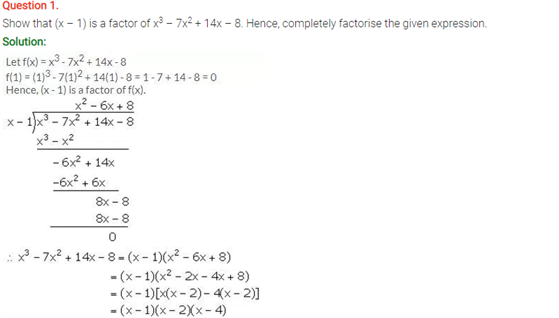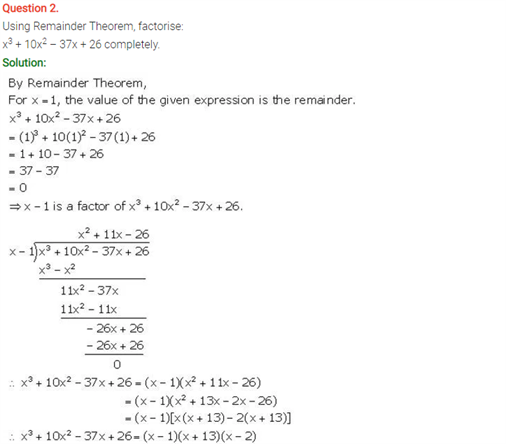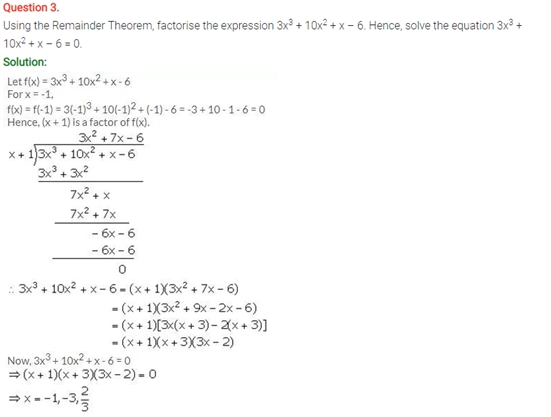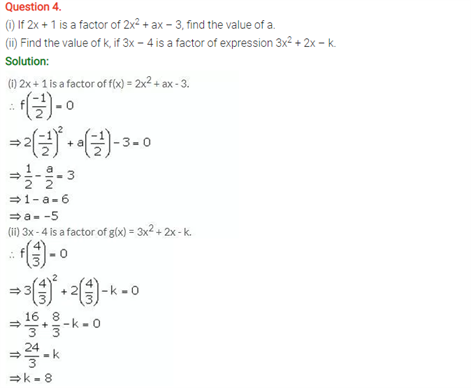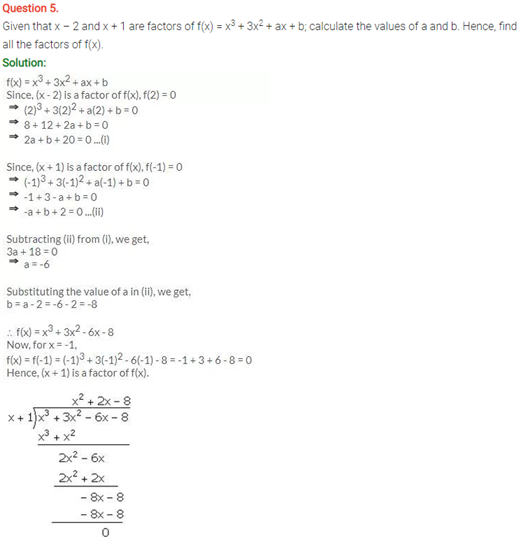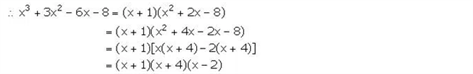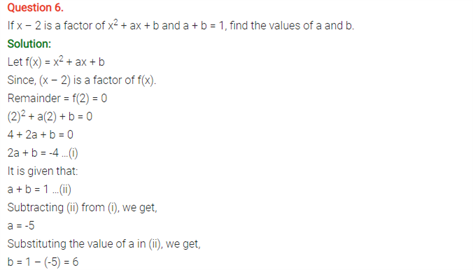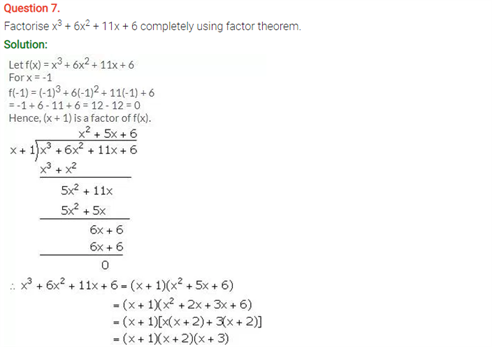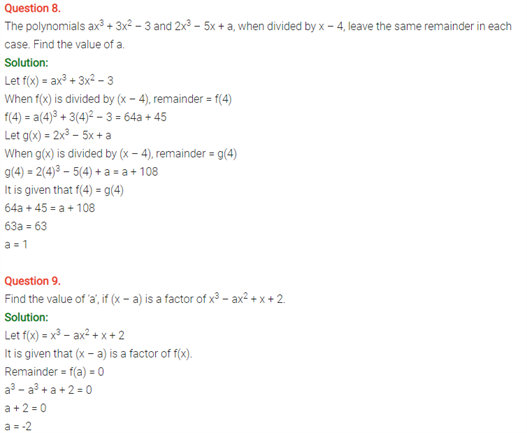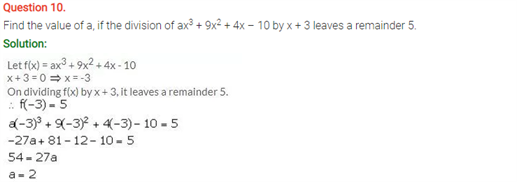# Selina Concise Maths Solution for ICSE Class 10 Chapter 8 Remainder and Factor Theorems

## Selina Class 10 Maths Solutions for Chapter 8

Here students will find some more questions on the Remainder and Factor Theorems. Students can find Important concepts on the ICSE Class 10 Maths Selina Solutions. For the need to understand the concepts in a better way, lets us see the most basic definitions.

### Explanation:

The remainder theorem tells us that for any polynomial f(x), if you divide it by the binomial x−a, the remainder is equal to the value of f(a). The factor theorem tells us that if a is a zero of a polynomial f(x), then (x−a) is a factor of f(x), and vice-versa.

For example, let’s consider the polynomial

f(x)=x2−2x+1

Using the remainder theorem

We can plug in 3 into f(x).

f(3)=32−2(3)+1

f(3)=9−6+1

f(3)=4

Therefore, by the remainder theorem, the remainder when you divide x2−2x+1by x−3 is 4.

You can also apply this in reverse. Divide x2−2x+1 by x−3, and the remainder you get is the value of f(3).

Using the factor theorem

The quadratic polynomial f(x)=x2−2x+1 equals 0 when x=1.

This tells us that (x−1) is a factor of x2−2x+1.

We can also apply the factor theorem in reverse:

We can factor x2−2x+1 into (x−1)2, therefore 1 is a zero of f(x).

Check out the ICSE Solutions Of Class 10 Maths Chapter 8 Remainder and Factor Theorems below: